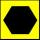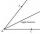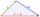# Triangle - 5th grade (10y) - examples

1. It is rectangular?Size of two of the angles in a triangle are: α=110°, β=40°. Is it a right triangle?
2. HexagonDraw a regular hexagon inscribed in a circle with radius r=8 cm. What is its perimeter?
3. TriangleProve whether you can construct a triangle ABC, if a=9 cm, b=10 cm, c=4 cm.
4. AngleDraw angle |∠ ABC| = 130° and built its axis. What angle is between axis angle and arm of angle?
5. TriangleDetermine if it is possible to construct a triangle with sides 28 31 34 by calculation.
6. Triangle ABCIn a triangle ABC side b measure 10 cm less than the side a and side b is half of the side c. Calculate the length of sides if the circumference of the triangle is 42 cm.
7. TrianglesIvo wants to draw all the triangles whose two sides of which have a length of 4 cm and 9 cm and the length of the third side is expressed in whole centimeters. How many triangles does he have?5 triangles + 1 square = how many sides in all